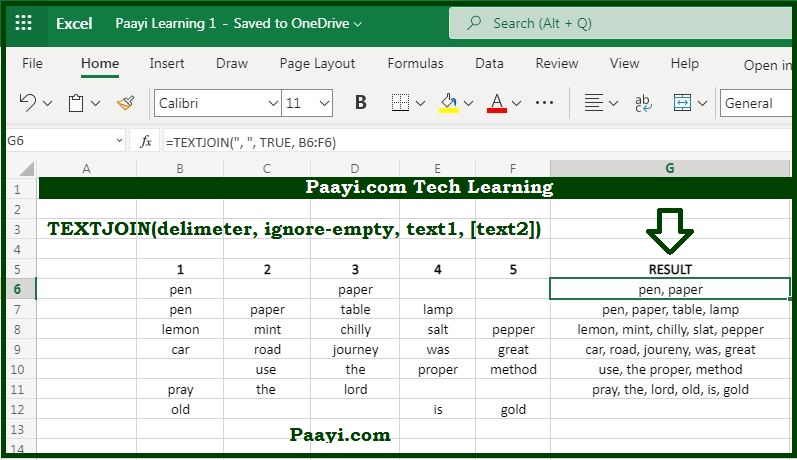# Learn How to Use Microsoft Excel TEXTJOIN Function

Written by | 0 Comments | 772 Views

In this article, you will learn how to use the Microsoft Excel TEXTJOIN function and its prime function in Microsoft Excel. You will also get to know the Microsoft Excel TEXTJOIN function return value and syntax with the help of some examples.

### Microsoft Excel TEXTJOIN Function

The prime function of the TEXTJOIN function is to join values with a delimiter. That means with the help of the Microsoft Excel TEXTJOIN function; you can able to join or concatenates values with the given delimiter. It is different from the CONCAT function and allows you to supply a range of cells and got a setting to ignore empty values.

### Microsoft Excel TEXTJOIN Function Return Value

It will return the joined text.

### Microsoft Excel TEXTJOIN Function Syntax

= TEXTJOIN(delimiter, ignore-empty, text1, [text2], ...)

Where the arguments:

• delimiter: It is the separator between each text.
• ignore-empty: If you want to ignore empty cells or not.
• text1: This is the first text value or range.
• text2: This is the second text value or range (Optional).

### How to Use the Microsoft Excel TEXTJOIN Function?The TEXTJOIN function is new and recently added in Office 365 and MS Excel 2019. Let us understand it with the examples provided below:

Example 1: To join cells from A1 to A3 with commas and spaces, you use the functions like this:

= TEXTJOIN(",  ", TRUE, A1:A3)

The return value will be whatever will be there in cell A1:A3 as - A1, A2, A3.

Example 2: If you want to join the cells A1:A3, without delimiter, including empty values, you can use the formula:

= TEXTJOIN(" ", FALSE, A1:A3)

This will return whatever will be there in the cells A1:A3 as - A1A2A3.